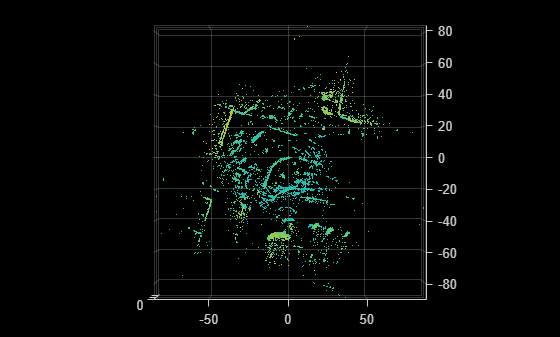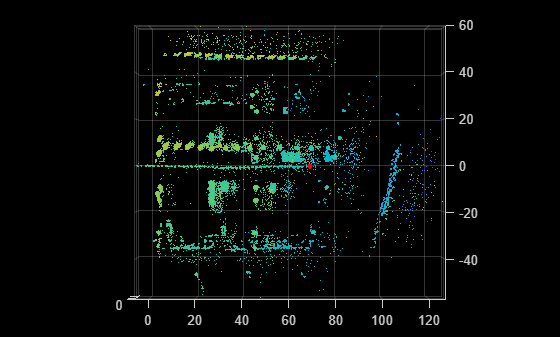# pcmapndt

Localization map based on normal distributions transform (NDT)

Since R2021a

## Description

The `pcmapndt` object creates a normal distributions transform (NDT) map from a prebuilt point cloud map of the environment. The NDT map is a compressed, memory-efficient representation suitable for localization. The object converts the point cloud map into a set of voxels (3-D boxes), each represented by a 3-D normal distribution. Use the `selectSubmap` object function to select a submap within the map from a coarse position estimate. Use the `findPose` object function to localize the pose of the sensor based on the assembled map.

## Creation

### Syntax

``ndtMap = pcmapndt(ptCloudMap,voxelSize)``

### Description

example

````ndtMap = pcmapndt(ptCloudMap,voxelSize)` returns an NDT map from a point cloud map, `ptCloudMap`.```

### Input Arguments

expand all

Point cloud map, specified as a `pointCloud` object. The `pcmapndt` object creates a normal distributions transform (NDT) map from the prebuilt map of the environment, specified by `ptCloudMap`.

Size of the voxels, specified as a scalar value in world units.

## Properties

expand all

Currently selected submap, specified as a 6-element vector of the form [xmin,xmax ymin ymax zmin zmax] that describes the range of the submap along each axis. The elements of the vector describe the region of interest represented by the submap.

Size of the voxels, specified as a scalar value in world units.

Range of the map along the x-axis, specified as a 2-element vector of the form [xmin xmax] .

Range of the map along the Y-axis, specified as a 2-element vector of the form [ymin ymax] .

Range of the map along the z-axis, specified as a 2-element vector of the form [zmin zmax] .

Mean value of each voxel, specified as an M-by-3 matrix. Each row of the matrix contains the [x y z] values for a voxel. M is the number of voxels.

Covariance of each voxel, specified as a 3-by-3-by-M array for M voxels.

Number of points in each voxel, specified as an M-by-1 vector for M voxels.

## Object Functions

 `selectSubmap` Select submap within map `isInsideSubmap` Check if query position is inside selected submap `findPose` Localize point cloud within map using normal distributions transform (NDT) algorithm `show` Visualize normal distributions transform (NDT) map

## Examples

collapse all

Load a point cloud view set, which was saved from a `pcviewset` object.

```data = load('vSetPointClouds.mat'); vSet = data.vSet;```

Extract point clouds and absolute poses to build a map.

```ptClouds = vSet.Views.PointCloud; tforms = vSet.Views.AbsolutePose;```

Create a point cloud map by aligning the point cloud scans using the absolute poses.

`ptCloudMap = pcalign(ptClouds,tforms);`

Create and visualize an NDT map from a point cloud map.

```voxelSize = 1; ndtMap = pcmapndt(ptCloudMap,voxelSize); figure show(ndtMap) view(2) % Change viewing angle to top-view```Load a normal distributions transform (NDT) map from a MAT file.

```data = load('ndtMapParkingLot.mat'); ndtMap = data.ndtMapParkingLot;```

Load point cloud scans and pose estimates from a second MAT file.

```data = load('parkingLotData.mat'); ptCloudScans = data.parkingLotData.ptCloudScans; initPoseEsts = data.parkingLotData.initPoseEsts;```

Display the NDT map.

`show(ndtMap)`

Change the viewing angle to top-view.

`view(2)`

Select the submap centered around the first estimate.

```center = initPoseEsts(1).Translation; sz = [70 50 20]; ndtMap = selectSubmap(ndtMap,center,sz);```

Set the radius for visualization of the current location and the distance threshold to update the submap.

```radius = 0.5; distThresh = 15;```

Loop over the point clouds, localize them in the map, and update the selected submap as needed.

```numScans = numel(ptCloudScans); for n = 1:numScans ptCloud = ptCloudScans(n); initPose = initPoseEsts(n); poseTranslation = initPose.Translation; [isInside,distToEdge] = isInsideSubmap(ndtMap,poseTranslation); submapNeedsUpdate = ~isInside ... % Current pose is outside submap || any(distToEdge(1:2) < distThresh); % Current pose is close to submap edge if submapNeedsUpdate ndtMap = selectSubmap(ndtMap,poseTranslation,sz); end % Localize the point cloud scan in the map. currPose = findPose(ndtMap,ptCloud,initPose); % Display the position of the estimate as a circle. pos = [currPose.Translation(1:2) radius]; showShape('circle',pos,'Color','r'); % Pause to view the change. pause(0.05) end```Biber, P., and W. Strasser. “The Normal Distributions Transform: A New Approach to Laser Scan Matching.” In Proceedings 2003 IEEE/RSJ International Conference on Intelligent Robots and Systems (IROS 2003) (Cat. No.03CH37453) Vol. 3, 2743–48. Las Vegas, Nevada, USA: IEEE, 2003. https://doi.org/10.1109/IROS.2003.1249285.

 Magnusson, Martin. "The Three-Dimensional Normal-Distributions Transform: An Efficient Representation for Registration, Surface Analysis, and Loop Detection." PhD thesis, Örebro universitet, 2009. http://urn.kb.se/resolve?urn=urn:nbn:se:oru:diva-8458 urn:nbn:se:oru:diva-8458.